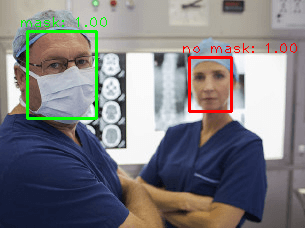# AIZOO Face Mask Detector using TensorFlow 2

Coronavirus (COVID-19) has affected many countries around the world. One of the protection method is to wear a face mask in public spaces. Many service providers and event organisers require customers to wear a mask. In this tutorial, we provide example how to use AIZOO face mask detector in TensorFlow 2.

AIZOO FaceMaskDetection is an open-source project provided on GitHub. This project is used to detect faces in an image and determine whether people are wearing mask or not.

In order to use AIZOO face mask detector we need to clone the repository.

``````git clone https://github.com/AIZOOTech/FaceMaskDetection.git

Using `pip` package manager install `tensorflow` and `opencv-python` from the command line.

``````pip install tensorflow
pip install opencv-python``````

OpenCV is used for image preprocessing. We read an image using `cv2.imread` function. The order of colors is BGR (Blue, Green, Red). However, algorithm requires the order of colors to be RGB (Red, Green, Blue). So we use `cv2.cvtColor` function to convert from BGR to RGB.

Model was trained using 260x260 images. We resize an image by using `cv2.resize` function. Model requires that shape of input will be `[batch, height, width, channels]`. We have an image which shape is `[height, width, channels]`. We add an `batch` dimension by passing `axis=0` to `numpy.expand_dims` function. We apply normalization on an image by dividing each pixel value by 255 to get a range of 0 to 1.

Repository contains pre-trained models. We load the model and do inference. Model is based on Single Shot Multibox Detector (SSD). We have two possible classes: `mask` and `no mask`. Model predicts offsets for each anchor box along with class scores. The actual bounding boxes according to the anchors are decoded by using `decode_bbox` function. The most appropriate bounding boxes are selected by using non-maximum suppression (NMS). Finally, bounding boxes along with class labels and scores are displayed on an image.

test.py

``````import numpy as np
import cv2
from utils.anchor_decode import decode_bbox
from utils.anchor_generator import generate_anchors
from utils.nms import single_class_non_max_suppression

imgPath = 'test.jpg'

HEIGHT = 260
WIDTH = 260

img = cv2.cvtColor(imgOriginal, cv2.COLOR_BGR2RGB)
img = cv2.resize(img, (WIDTH, HEIGHT))
img = np.expand_dims(img, axis=0) / 255

session, graph = load_tf_model(modelPath)
anchorOffsets, scores = tf_inference(session, graph, img)

maxScores = np.max(scores, axis=1)
maxScoreLabels = np.argmax(scores, axis=1)

featureMapSizes = [[33, 33], [17, 17], [9, 9], [5, 5], [3, 3]]
anchorSizes = [[0.04, 0.056], [0.08, 0.11], [0.16, 0.22], [0.32, 0.45], [0.64, 0.72]]
anchorRatios = [[1, 0.62, 0.42]] * 5

anchors = generate_anchors(featureMapSizes, anchorSizes, anchorRatios)
anchors = np.expand_dims(anchors, axis=0)
boxes = decode_bbox(anchors, anchorOffsets)

selectedIdxs = single_class_non_max_suppression(boxes, maxScores,
conf_thresh=0.5, iou_thresh=0.4)

height, width, _ = imgOriginal.shape
for idx in selectedIdxs:
score = float(maxScores[idx])
predictedLabel = maxScoreLabels[idx]

box = boxes[idx]
xmin = max(0, int(box * width))
ymin = max(0, int(box * height))
xmax = min(int(box * width), width)
ymax = min(int(box * height), height)

if predictedLabel == 0:
color = (0, 255, 0)
else:
color = (0, 0, 255)

cv2.rectangle(imgOriginal, (xmin, ymin), (xmax, ymax), color, thickness=2)

text = '%s: %.2f' % (classNames[predictedLabel], score)
cv2.putText(imgOriginal, text, (xmin - 8, ymin - 4), cv2.FONT_HERSHEY_PLAIN, 1, color)

cv2.imshow('Face mask detection', imgOriginal)
cv2.waitKey(0)``````

The image for testing was taken from MAFA (MAsked FAces) dataset.